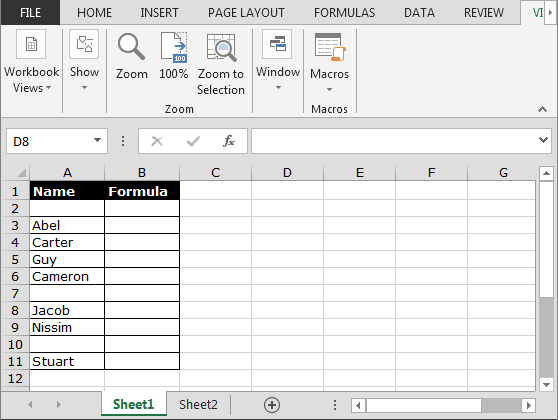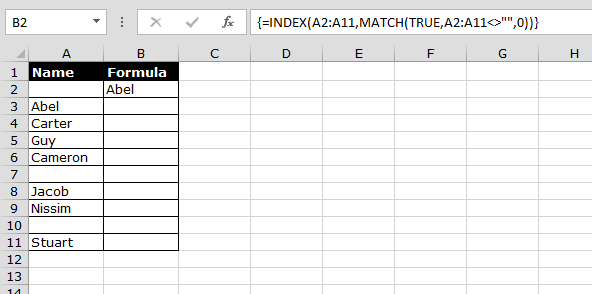# Retrieving the Value of the First Nonblank Cell in a List

To retrieve the value of the first non blank cell in a list, we will use Index and Match functions.

Why and how we use Index and Match functions together to retrieve the first nonblank value from a range?

Index formula returns a value from the intersection between the row number and the column number in an Array. In this situation, Index formula will help to pick the value.

Match returns the cell number where the value is found in a horizontal or vertical range. In this situation, Match function will help to match the provided value in the range.

Let’s take an example to understand how we can retrieve value of first non-blank cell.

We have data in Excel in which column A contains the name. Now, we want to retrieve the first nonblank name in cell B2.• Enter the formula in cell B2.
• =INDEX(A2:A11,MATCH(TRUE,A2:A11<>"",0))
• This is an array formula. So don’t press only Enter.
• Press Ctrl+Shift+Enter.Now we can see that the formula has returned first non blank cell.

This is the way we can use Index and Match functions to retrieve the first non-blank cell in a list.